# Charting

## Bokeh

### Introduction

bokeh is a Python library you can use to create interactive visualizations. It helps you build beautiful graphics, ranging from simple plots to complex dashboards with streaming datasets. With bokeh, you can create JavaScript-powered visualizations without writing any JavaScript.

### Import Libraries

Follow these steps to import the libraries that you need:

1. Import the bokeh library.
2. from bokeh.plotting import figure, show
from bokeh.models import BasicTicker, ColorBar, ColumnDataSource, LinearColorMapper
from bokeh.palettes import Category20c
from bokeh.transform import cumsum, transform
from bokeh.io import output_notebook
3. Call the output_notebook method.
4. output_notebook()
5. Import the numpy library.
6. import numpy as np

### Get Historical Data

Get some historical market data to produce the plots. For example, to get data for a bank sector ETF and some banking companies over 2021, run:

qb = QuantBook()
tickers = ["XLF",   # Financial Select Sector SPDR Fund
"COF",   # Capital One Financial Corporation
"GS",    # Goldman Sachs Group, Inc.
"JPM",   # J P Morgan Chase & Co
"WFC"]   # Wells Fargo & Company
symbols = [qb.AddEquity(ticker, Resolution.Daily).Symbol for ticker in tickers]
history = qb.History(symbols, datetime(2021, 1, 1), datetime(2022, 1, 1))

### Create Candlestick Chart

You must import the plotting libraries and get some historical data to create candlestick charts.

In this example, you create a candlestick chart that shows the open, high, low, and close prices of one of the banking securities. Follow these steps to create the candlestick chart:

1. Select a Symbol.
2. symbol = symbols
3. Slice the history DataFrame with the symbol.
4. data = history.loc[symbol]
5. Divide the data into days with positive returns and days with negative returns.
6. up_days = data[data['close'] > data['open']]
down_days = data[data['open'] > data['close']]
7. Call the figure function with a title, axis labels and x-axis type.
8. plot = figure(title=f"{symbol} OHLC", x_axis_label='Date', y_axis_label='Price', x_axis_type='datetime')
9. Call the segment method with the data timestamps, high prices, low prices, and a color.
10. plot.segment(data.index, data['high'], data.index, data['low'], color="black")

This method call plots the candlestick wicks.

11. Call the vbar method for the up and down days with the data timestamps, open prices, close prices, and a color.
12. width = 12*60*60*1000
plot.vbar(up_days.index, width, up_days['open'], up_days['close'],           fill_color="green", line_color="green")
plot.vbar(down_days.index, width, down_days['open'], down_days['close'],          fill_color="red", line_color="red")

This method call plots the candlestick bodies.

13. Call the show function.
14. show(plot)

The Jupyter Notebook displays the candlestick chart.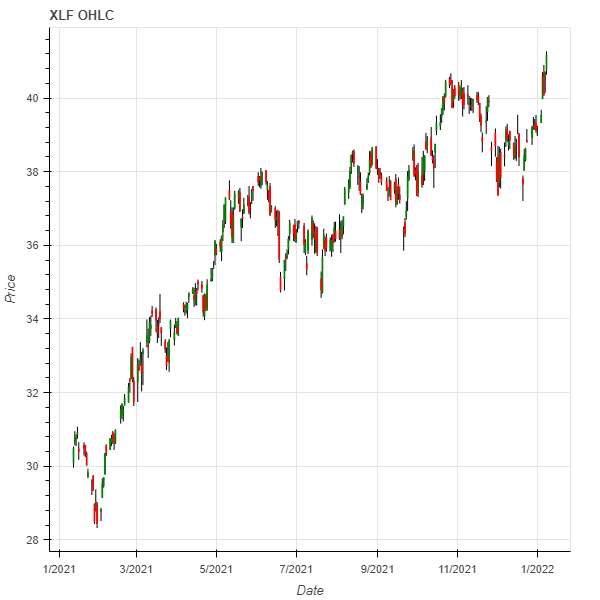### Create Line Plot

You must import the plotting libraries and get some historical data to create line charts.

In this example, you create a line chart that shows the closing price for one of the banking securities. Follow these steps to create the line chart:

1. Select a Symbol.
2. symbol = symbols
3. Slice the history DataFrame with the symbol and then select the close column.
4. close_prices = history.loc[symbol]['close']
5. Call the figure function with title, axis labels and x-axis type..
6. plot = figure(title=f"{symbol} Close Price", x_axis_label='Date', y_axis_label='Price', x_axis_type='datetime')
7. Call the line method with the timestamps, close_prices, and some design settings.
8. plot.line(close_prices.index, close_prices,
legend_label=symbol.Value, color="blue", line_width=2)
9. Call the show function.
10. show(plot)

The Jupyter Notebook displays the line plot.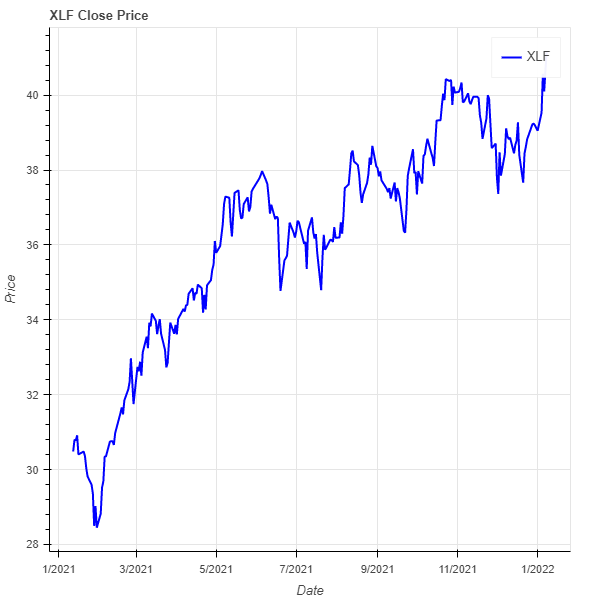### Create Scatter Plot

You must import the plotting libraries and get some historical data to create scatter plots.

In this example, you create a scatter plot that shows the relationship between the daily returns of two banking securities. Follow these steps to create the scatter plot:

1. Select 2 Symbols.
2. For example, to select the Symbols of the first 2 bank stocks, run:

symbol1 = symbols
symbol2 = symbols
3. Slice the history DataFrame with each Symbol and then select the close column.
4. close_price1 = history.loc[symbol1]['close']
close_price2 = history.loc[symbol2]['close']
5. Call the pct_change and dropna methods on each Series.
6. daily_return1 = close_price1.pct_change().dropna()
daily_return2 = close_price2.pct_change().dropna()
7. Call the polyfit method with the daily_returns1, daily_returns2, and a degree.
8. m, b = np.polyfit(daily_returns1, daily_returns2, deg=1)

This method call returns the slope and intercept of the ordinary least squares regression line.

9. Call the linspace method with the minimum and maximum values on the x-axis.
10. x = np.linspace(daily_returns1.min(), daily_returns1.max())
11. Calculate the y-axis coordinates of the regression line.
12. y = m*x + b
13. Call the figure function with a title and axis labels.
14. plot = figure(title=f"{symbol1} vs {symbol2} Daily Return",
x_axis_label=symbol1.Value, y_axis_label=symbol2.Value)
15. Call the line method with x- and y-axis values, a color, and a line width.
16. plot.line(x, y, color="red", line_width=2)

This method call plots the regression line.

17. Call the dot method with the daily_returns1, daily_returns2, and some design settings.
18. plot.dot(daily_returns1, daily_returns2, size=20, color="navy", alpha=0.5)

This method call plots the scatter plot dots.

19. Call the show function.
20. show(plot)

The Jupyter Notebook displays the scatter plot.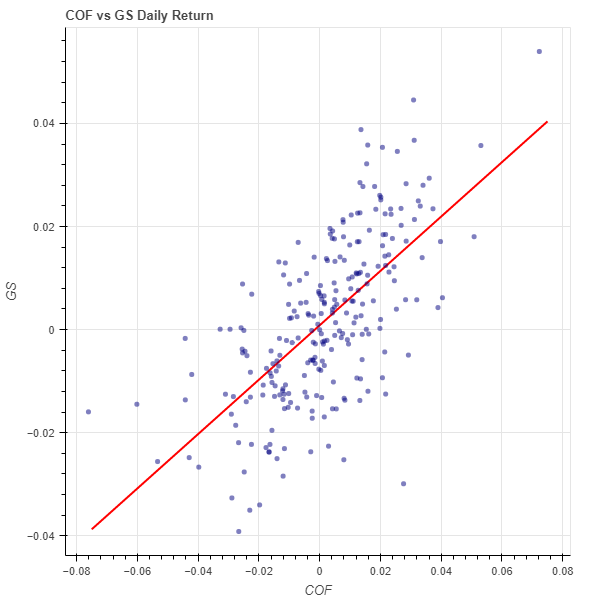### Create Histogram

You must import the plotting libraries and get some historical data to create histograms.

In this example, you create a histogram that shows the distribution of the daily percent returns of the bank sector ETF. In addition to the bins in the histogram, you overlay a normal distribution curve for comparison. Follow these steps to create the histogram:

1. Select the Symbol.
2. symbol = symbols
3. Slice the history DataFrame with the symbol and then select the close column.
4. close_prices = history.loc[symbol]['close']
5. Call the pct_change method and then call the dropna method.
6. daily_returns = close_prices.pct_change().dropna()
7. Call the histogram method with the daily_returns, the density argument enabled, and a number of bins.
8. hist, edges = np.histogram(daily_returns, density=True, bins=20)

This method call returns the following objects:

• hist: The value of the probability density function at each bin, normalized such that the integral over the range is 1.
• edges: The x-axis value of the edges of each bin.
9. Call the figure method with a title and axis labels.
10. plot = figure(title=f"{symbol} Daily Return Distribution",
x_axis_label='Return', y_axis_label='Frequency')
11. Call the quad method with the coordinates of the bins and some design settings.
12. plot.quad(top=hist, bottom=0, left=edges[:-1], right=edges[1:],
fill_color="navy", line_color="white", alpha=0.5)

This method call plots the histogram bins.

13. Call the mean and std methods.
14. mean = daily_returns.mean()
std = daily_returns.std()
15. Call the linspace method with the lower limit, upper limit, and number data points for the x-axis of the normal distribution curve.
16. x = np.linspace(-3*std, 3*std, 1000)
17. Calculate the y-axis values of the normal distribution curve.
18. pdf = 1/(std * np.sqrt(2*np.pi)) * np.exp(-(x-mean)**2 / (2*std**2))
19. Call the line method with the data and style of the normal distribution PDF curve.
20. plot.line(x, pdf, color="red", line_width=4,
alpha=0.7, legend_label="Normal Distribution PDF")

This method call plots the normal distribution PDF curve.

21. Call show to show the plot.
22. show(plot)

The Jupyter Notebook displays the histogram.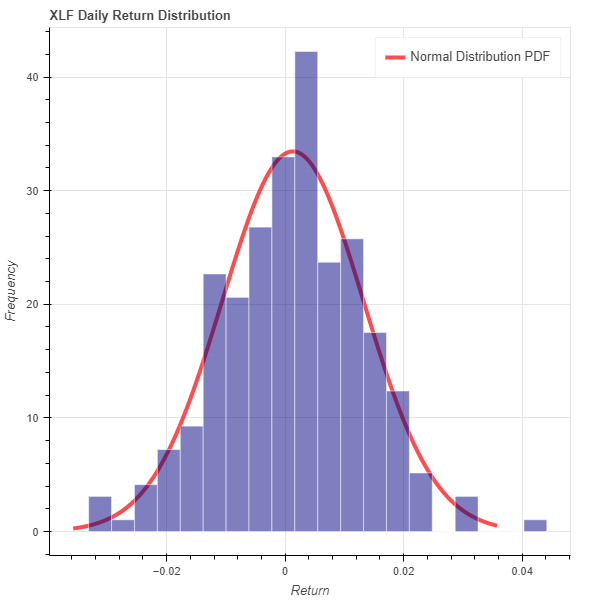### Create Bar Chart

You must import the plotting libraries and get some historical data to create bar charts.

In this example, you create a bar chart that shows the average daily percent return of the banking securities. Follow these steps to create the bar chart:

1. Select the close column and then call the unstack method.
2. close_prices = history['close'].unstack(level=0)
3. Call the pct_change method and then multiply by 100.
4. daily_returns = close_prices.pct_change() * 100
5. Call the mean method.
6. avg_daily_returns = daily_returns.mean()
7. Call the DataFrame constructor with the data Series and then call the reset_index method.
8. avg_daily_returns = pd.DataFrame(avg_daily_returns, columns=['avg_return']).reset_index()
9. Call the figure function with a title, x-axis values, and axis labels.
10. plot = figure(title='Banking Stocks Average Daily % Returns', x_range=avg_daily_returns['symbol'],
x_axis_label='%', y_axis_label='Stocks')
11. Call the vbar method with the avg_daily_returns, x- and y-axis column names, and a bar width.
12. plot.vbar(source=avg_daily_returns, x='symbol', top='avg_return', width=0.8)
13. Rotate the x-axis label and then call the show function.
14. plot.xaxis.major_label_orientation = 0.6
show(plot)

The Jupyter Notebook displays the bar chart.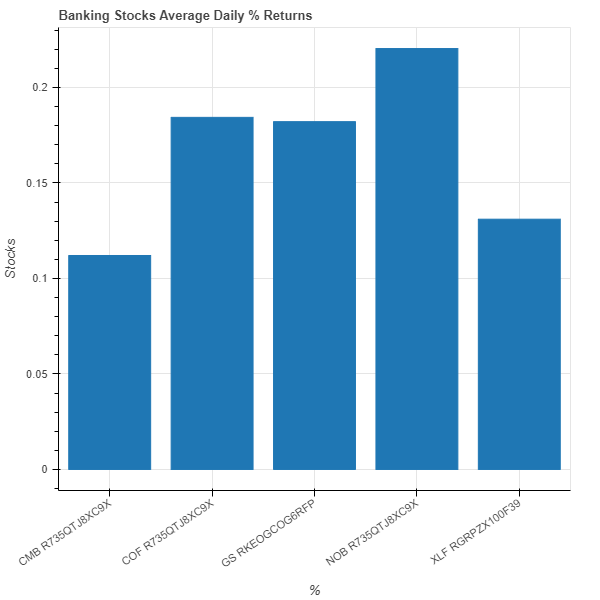### Create Heat Map

You must import the plotting libraries and get some historical data to create heat maps.

In this example, you create a heat map that shows the correlation between the daily returns of the banking securities. Follow these steps to create the heat map:

1. Select the close column and then call the unstack method.
2. close_prices = history['close'].unstack(level=0)
3. Call the pct_change method.
4. daily_returns = close_prices.pct_change()
5. Call the corr method.
6. corr_matrix = daily_returns.corr()
7. Set the index and columns of the corr_matrix to the ticker of each security and then set the name of the column and row indices.
8. corr_matrix.index = corr_matrix.columns = [symbol.Value for symbol in symbols]
corr_matrix.index.name = 'symbol'
corr_matrix.columns.name = "stocks"
9. Call the stack, rename, and reset_index methods.
10. corr_matrix = corr_matrix.stack().rename("value").reset_index()
11. Call the figure function with a title, axis ticks, and some design settings.
12. plot = figure(title=f"Banking Stocks and Bank Sector ETF Correlation Heat Map",
x_range=list(corr_matrix.symbol.drop_duplicates()),
y_range=list(corr_matrix.stocks.drop_duplicates()),
toolbar_location=None,
tools="",
x_axis_location="above")
13. Select a color palette and then call the LinearColorMapper constructor with the color pallet, the minimum correlation, and the maximum correlation.
14. colors = Category20c[len(corr_matrix.columns)]
mapper = LinearColorMapper(palette=colors, low=corr_matrix.value.min(),
high=corr_matrix.value.max())
15. Call the rect method with the correlation plot data and design setting.
16. plot.rect(source=ColumnDataSource(corr_matrix),
x="stocks",
y="symbol",
width=1,
height=1,
line_color=None,
fill_color=transform('value', mapper))
17. Call the ColorBar constructor with the mapper, a location, and a BaseTicker.
18. color_bar = ColorBar(color_mapper=mapper,
location=(0, 0),
ticker=BasicTicker(desired_num_ticks=len(colors)))

This snippet creates a color bar to represent the correlation coefficients of the heat map cells.

19. Call the add_layout method with the color_bar and a location.
20. plot.add_layout(color_bar, 'right')

This method call plots the color bar to the right of the heat map.

21. Call the show function.
22. show(plot)

The Jupyter Notebook displays the heat map.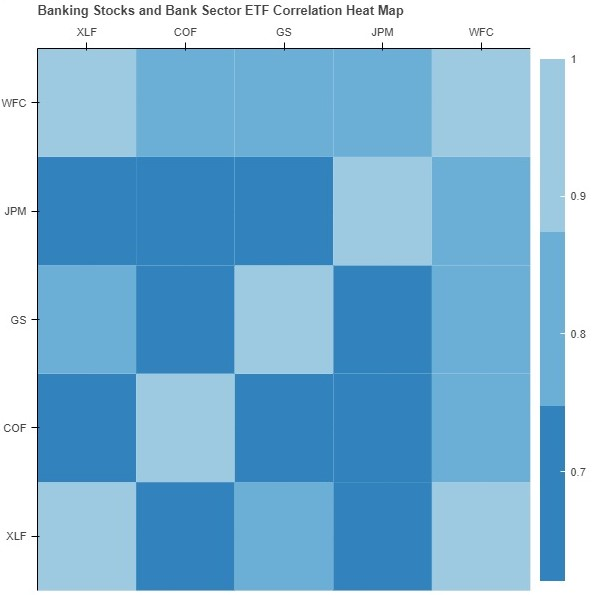### Create Pie Chart

You must import the plotting libraries and get some historical data to create pie charts.

In this example, you create a pie chart that shows the weights of the banking securities in a portfolio if you allocate to them based on their inverse volatility. Follow these steps to create the pie chart:

1. Select the close column and then call the unstack method.
2. close_prices = history['close'].unstack(level=0)
3. Call the pct_change method.
4. daily_returns = close_prices.pct_change()
5. Call the var method, take the inverse, and then normalize the result.
6. inverse_variance = 1 / daily_returns.var()
inverse_variance /= np.sum(inverse_variance)   # Normalization
inverse_variance *= np.pi*2    # For a full circle circumference in radian
7. Call the DataFrame constructor with the inverse_variance Series and then call the reset_index method.
8. inverse_variance = pd.DataFrame(inverse_variance, columns=["inverse variance"]).reset_index()
9. Add a color column to the inverse_variance DataFrame.
10. inverse_variance['color'] = Category20c[len(inverse_variance.index)]
11. Call the figure function with a title.
12. plot = figure(title=f"Banking Stocks and Bank Sector ETF Allocation")
13. Call the wedge method with design settings and the inverse_variance DataFrame.
14. plot.wedge(x=0, y=1, radius=0.6, start_angle=cumsum('inverse variance', include_zero=True),
end_angle=cumsum('inverse variance'), line_color="white", fill_color='color',
legend_field='symbol', source=inverse_variance)
15. Call the show function.
16. show(plot)

The Jupyter Notebook displays the pie chart.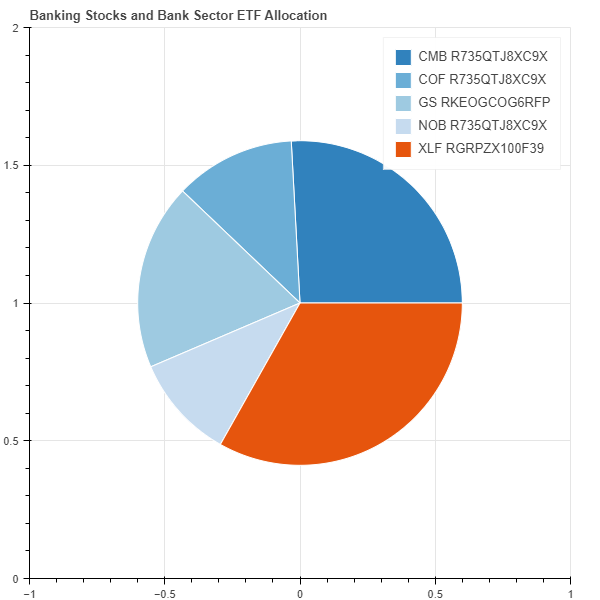You can also see our Videos. You can also get in touch with us via Discord.